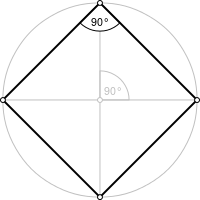## Calculate Perimeter Of SquarePerimeter = 4 x Length

 Enter your values: Length: Results: Perimeter Of a Square:

In geometry, a square is a regular quadrilateral, which means that it has four equal sides and four equal angles (90-degree angles, or right angles). It can also be defined as a rectangle in which two adjacent sides have equal length.

Thinkcalculator.com provides you helpful and handy calculator resources.